Installation

devtools::install_github("Marie-PerrotDockes/BlockCov")

Introduction

This package implements the algorithm proposed by @blc. For further details we refer the reader to this paper. We shall consider the following framework. Let $$\boldsymbol{E}_1, \boldsymbol{E}_2,\cdots,\boldsymbol{E}_n$$, $$n$$ zero-mean i.i.d. $$q$$-dimensional random vectors having a covariance matrix $$\boldsymbol{\Sigma}$$ such that the number $$q$$ of its rows and columns is much larger than $$n$$. The goal of the package is to propose a new estimator of $$\boldsymbol{\Sigma}$$ and of the square root of its inverse in the particular case where $$\boldsymbol{\Sigma}$$ is assumed to have a block structure without limiting ourselves to diagonal blocks. More precisely, in this paper, we shall assume that

$\boldsymbol{\Sigma}=\boldsymbol{Z}\;\boldsymbol{Z}‘+\boldsymbol{D},$

where $$\boldsymbol{Z}$$ is a $$q \times k$$ sparse matrix with $$k\ll q$$, $$\boldsymbol{Z}’$$ denotes the transpose of the matrix $$\boldsymbol{Z}$$ and $$\boldsymbol{D}$$ is a diagonal matrix such that the diagonal terms of $$\boldsymbol{\Sigma}$$ are equal to one.

Our approach consists in providing a low rank matrix approximation of the $$\boldsymbol{Z}\;\boldsymbol{Z}‘$$ part of $$\boldsymbol{\Sigma}$$ and then in using a $$\ell_1$$ regularization in order to obtain a sparse estimator of $$\boldsymbol{\Sigma}$$. More precisely, since $$\boldsymbol{\Sigma}$$ is a correlation matrix, it is a symmetric matrix with ones on its diagonal, thus all the information is contained in its upper triangular part without its diagonal. If we know $$\boldsymbol{P}$$ the $$(q-1)\times (q-1)$$ symmetric matrix, which has for upper triangular part the upper triangular part of $$\boldsymbol{\Sigma}$$ without its diagonal, we know $$\boldsymbol{\Sigma}$$. The matrix $$\boldsymbol{P}$$ has the advantage to have a low rank. In the following, we propose to first estimate the block matrix $$\boldsymbol{P}$$.

We shall moreover propose a methodology to estimate $$\boldsymbol{\Sigma}$$ in the case where the block structure is latent that is when the columns and rows of $$\boldsymbol{\Sigma}$$ have to be permuted according to an unknown permutation in order to make the block structure appear. In this case, a hierarchical clustering step has to be applied beforehand.

Simulation of $$\boldsymbol{\Sigma}$$ having a block structure

In order to generate a matrix $$\boldsymbol{\Sigma}$$ having a block structure with extra-diagonal blocks and $$q=100$$, we can use the function |Simu_Sigma| as follows:

q <- 100
Sigma <- Simu_Sigma(q = q, diag = FALSE, equal = TRUE)

The matrix $$\boldsymbol{\Sigma}$$ is displayed in Figure \ref{fig:fig0}.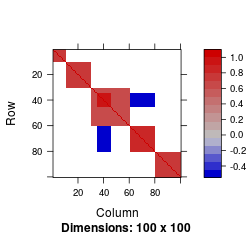Using the matrix $$\boldsymbol{\Sigma}$$ generated by the function |Simu_Sigma| a $$n\times q$$ matrix $$\boldsymbol{E}$$ was generated such that its rows are independent zero-mean Gaussian random vectors having a covariance matrix equal to $$\boldsymbol{\Sigma}$$ and $$n=30$$.

n <- 30
E <- matrix(rnorm(n * q), ncol = q) %*% chol(as.matrix(Sigma))

Estimation of $$\boldsymbol{\Sigma}$$ (without estimating and $$\boldsymbol{\Sigma}^{-½}$$)

Estimation of $$\boldsymbol{\Sigma}$$ when the parameters are known

In order to get an estimator of $$\boldsymbol{\Sigma}$$ the function |Sigma_estimation| was applied. Since the data set was simulated, the rank of $$\boldsymbol{P}$$, the sub-matrix of $$\boldsymbol{\Sigma}$$, and its number of non null values are known.

k <- 5
nb_nn0 <- sum(Sigma[upper.tri(Sigma, diag = FALSE)] != 0)
res_known <-  Sigma_estimation(E, k = k, nb_nn0 = nb_nn0)

Our estimator $\widehat{\boldsymbol{\Sigma}}$ of $$\boldsymbol{\Sigma}$$ is given by |res_known$Sigma_est|. It is displayed in Figure \ref{fig:fig1} and is obtained by using: Matrix::image(res_known$Sigma_est)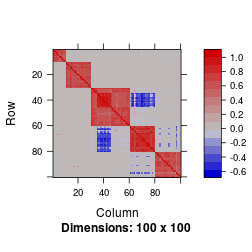The Frobenius norm $$\|\boldsymbol{\Sigma}-\widehat{\boldsymbol{\Sigma}}\|$$ is equal to 5.5.

For comparison purpose, the sample correlation matrix is displayed in Figure \ref{fig:fig2}.

Matrix::image(Matrix::Matrix(cor(E)))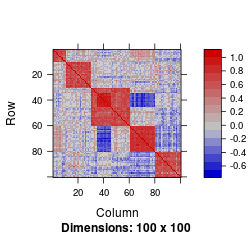The Frobenius norm $$\|\boldsymbol{\Sigma}-\widehat{\boldsymbol{\Sigma}}_{\textrm{emp}}\|$$ is equal to 14.5, where $$\widehat{\boldsymbol{\Sigma}}_{\textrm{emp}}$$ denotes the sample correlation matrix.

Estimation of $$\boldsymbol{\Sigma}$$ when the parameters are unknown

In practice, the number of non null values and the rank of $$\boldsymbol{P}$$ are unknown. These parameters can be both estimated using the function |Sigma_estimation|.

For choosing the rank of $$\boldsymbol{P}$$, two strategies are available in the package and compared in @blc:

• The first strategy is the \textsf{Cattell} criterion based on the Cattell's scree plot described in @cattell1966. In the package, it can be used by setting \verb|method_k = “Cattell”| in the \verb|Sigma_estimation| function.
• The second strategy is the \textsf{PA} permutation method proposed by @Horn1965. In the package, it can be used by setting \verb|method_k = “PA”| in the \verb|Sigma_estimation| function.

To choose the number of non null values two methodologies are also available in the package and compared in @blc:

• The \textsf{Elbow} method described in @blc. In the package, it can be used by setting \verb|method_0 = “Elbow”| in \verb|Sigma_estimation| function and
• the \textsf{BL} approach proposed in @bickel2008 based on cross-validation. In the package, it can be used by setting \verb|method_0 = “BL”| in the \verb|Sigma_estimation| function.

For example, an estimator of $$\boldsymbol{\Sigma}$$ using the \textsf{Cattell} criterion and the \textsf{Elbow} method can be obtained by using the |Sigma_estimation| function as follows:

res <-Sigma_estimation(E, method_k = "Cattell", method_0 = "Elbow")

It has to be noticed that “Cattell” and “Elbow” are the default value for |method_k| and |method_0| respectively. Hence, the same result can be obtain by using :

res <-Sigma_estimation(E)

The corresponding estimator of $$\boldsymbol{\Sigma}$$ is displayed in Figure \ref{fig:fig3}. The estimated rank and the estimated number of non null values can be obtained by |res$k| and |res$nb_nn0|, respectively. Here, the estimated rank is equal to 5 and the estimated number of non null values is 1155. Note that the true values of these parameters are 5 and 1270.

An estimator of $$\boldsymbol{\Sigma}$$ using the \textsf{PA} criterion and the \textsf{BL} method can be obtained by using the \verb|Sigma_estimation| function as follows:

res_pabl <- Sigma_estimation(E, method_k = "PA", method_0 = "BL")

The corresponding estimator of $$\boldsymbol{\Sigma}$$ is displayed in Figure \ref{fig:fig3pabl}. Here, the estimated rank is equal to 5 and the estimated number of non null values is 1146.

This second approach is a little bit slower than the first one especially for large values of $$q$$ but can be more accurate when the number of samples is large enough, see @blc for further details on the numerical and statistical performance of the different strategies.

Matrix::image(res$Sigma_est)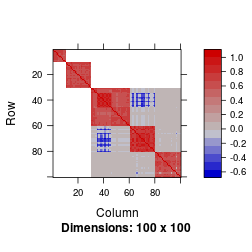Matrix::image(res_pabl$Sigma_est)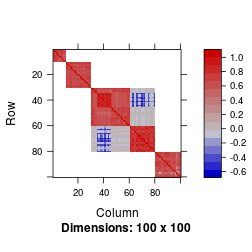We can see from this figure that the estimation of $$\boldsymbol{\Sigma}$$ does not seem to be altered by having to estimate the number of non null values and the rank of the matrix. The Frobenius norm $$\|\boldsymbol{\Sigma}-\widehat{\boldsymbol{\Sigma}}\|$$ is equal to 7.1 for the first estimator and to 7.3 for the second one.

An estimator of $$\boldsymbol{\Sigma}^{-½}$$ can be obtained using the \verb|Sigma_estimation| function by setting the arguments \verb|res$S_inv_12| to true as follows: res_both <- Sigma_estimation(E, method_k = "Cattell", method_0 = "Elbow", inv_12 = TRUE) An estimator of $$\boldsymbol{\Sigma}$$ is obtained with \verb|res_both$Sigma_est| and an estimator of $$\boldsymbol{\Sigma}^{-½}$$ is obtained with \verb|res_both$S_inv_12|. It can be used to remove the dependence that may exist between the columns of $$\boldsymbol{E}$$. Estimation of $$\boldsymbol{\Sigma}$$ and $$\boldsymbol{\Sigma}^{-½}$$ when the block structure is latent In practice, it is possible that the block structure of $$\boldsymbol{\Sigma}$$ only appears after having permuted its rows and columns according to a well chosen permutation. We explain hereafter how to estimate $$\boldsymbol{\Sigma}$$ and $$\boldsymbol{\Sigma}^{-½}$$ in this case. We first generate such a matrix by applying a random permutation to the rows and columns of the matrix $$\boldsymbol{\Sigma}$$ previously generated. samp <- sample(1:q, q, replace = FALSE) Sigma_samp <- Sigma[samp, samp] The corresponding matrix is displayed in Figure \ref{fig:fig4}. Matrix::image(Sigma_samp)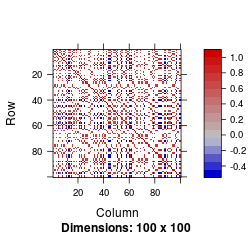In such a situation where the columns and rows have to be permuted according to an unknown permutation, we propose to use a hierarchical clustering as the first step of our methodology and then use the same strategy. This is performed by putting \verb|reorder=TRUE| in the arguments of the function \verb|Sigma_estimation|. E <- matrix(rnorm(n * q), ncol = q) %*% chol(as.matrix(Sigma_samp)) res_samp <- Sigma_estimation(E, reorder = TRUE, inv_12 = TRUE) The estimated matrix is displayed in Figure \ref{fig:fig5}. Matrix::image(res_samp$Sigma_est)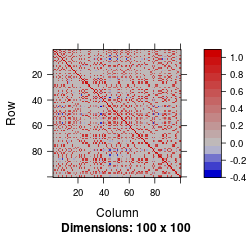The permutation to make the block structure appear is available from \verb|res_samp$order|. The corresponding estimated correlation matrix in which the columns have been permuted in order to make the block structure appear is obtained using the following lines and is displayed in Figure \ref{fig:fig6}: ord <- res_samp$order
Matrix::image(res_samp$Sigma_est[ord, ord])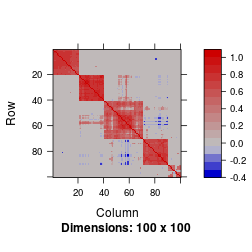This matrix has to be compared with the following one displayed in Figure \ref{fig:fig7}: Matrix::image(Sigma_samp[ord, ord])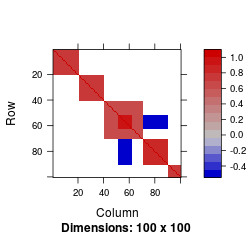Once again, our strategy does not seem to be altered by the permutation of the columns of the original matrix $$\boldsymbol{\Sigma}$$. The Frobenius norm of the error is equal to 12.4. In this situation $$\widehat{\boldsymbol{\Sigma}}^{-½}$$ is still available. The matrix $$\widehat{\boldsymbol{\Sigma}}^{-½}\boldsymbol{\Sigma}\widehat{\boldsymbol{\Sigma}}^{-½}$$, which is displayed in Figure \ref{fig:fig8}, should be close to the identity matrix: Matrix::image(res_samp$S_inv_12 %*% Sigma_samp %*%res_samp\$S_inv_12)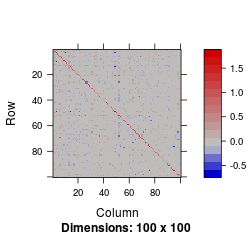The associated Frobenius norm $$||\widehat{\boldsymbol{\Sigma}}^{-½}\boldsymbol{\Sigma}\widehat{\boldsymbol{\Sigma}}^{-½}-\textrm{Id}_q||=$$ 7.8.

All the values of the Frobenius norms are quite close meaning that our methodology is efficient even when the parameters are unknown and when the columns and rows have to be permuted in order to make the block structure appear.